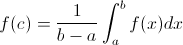# Test Prep Plan - Take a practice test

Take this practice test to check your existing knowledge of the course material. We'll review your answers and create a Test Prep Plan for you based on your results.
How Test Prep Plans work
1
2Based on your results, we'll create a customized Test Prep Plan just for you!
3Study smarter
Study more effectively: skip concepts you already know and focus on what you still need to learn.

# Calculus: Homework Help Resource Final Exam

Free Practice Test Instructions:

Choose your answer to the question and click 'Continue' to see how you did. Then click 'Next Question' to answer the next question. When you have completed the free practice test, click 'View Results' to see your results. Good luck!

#### Question 1 1. What is the equation for the area between f(x) and the x-axis from x=0 to x=2?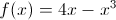#### Question 2 2. The lines a and b are parallel lines of length 5 and 2. The lines d and e are parallel to one another, and both are perpendicular to a and b. What is the length of c?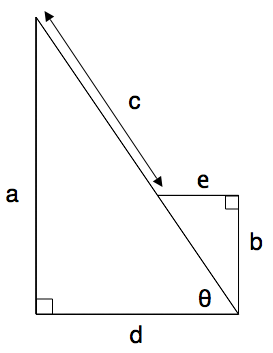#### Question 3 3. Use the given formula to solve for z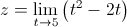#### Question 4 4. Calculate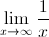#### Question 6 6. Using the squeeze theorem, what is z?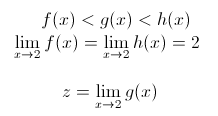#### Question 7 7. Use the graph and the integral to find z.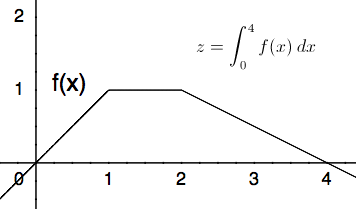#### Question 9 9. Find the derivative of f(x).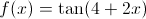#### Question 11 11. What are the regions of continuity for y defined as?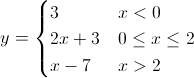#### Question 14 14. Integrate the following.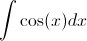#### Question 15 15. Given a continuous function f(x) that satisfies the equation below, which of the following is true, according to the average value theorem?